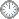# “礼”和“乐”的制约

This is an in-mail from TYUST.
新入の者--> What is going on ? (redirected)new

(接前: 16 15 14)  Theorem 2.13
.
(XF, BΦ) lc, -(Kx + B) nef ==> 存在 n-补 Kx + B (B ≥ B).

.

1) n-补 存在的充分条件;
2) n 和 B 的大体构造.
.

----  (X,  B) lc 是Fano理论的“标配”.
---- -(Kx + B) 是反运算形, 此处属性为 nef.

---- XF 指代 X 为 “Fano type”, 即对某个边界构成 klt弱Fano
---- BΦ指代 B ∈ Φ(R), 即B的系数属于Φ(R).
.

---- R 在文中为哥特式字体, 系 [0, 1] 内有限个有理数的集合.
---- Φ(R) 是形如 1 - r/m 的元素构成的集合, r ∈ R, m ∈ lN.
---- I(R) 是使得 Ir 为整数的最小自然数(对每个 r ∈ R).
---- 要求 n 被 I(R) 整除.

.

I(R) 是受约束的自然数, 暂称为“乐”.
(“礼” 和 “乐” 都建立在 R 之上).
.

---- B ≥ B 体现了 B 的构造 (即 B 以 B 一部分, 后者的系数属于Φ(R)).
---- n 被 I(R) 整除, 体现了 n 的构造 (即 n 至少以 I(R) 为因子).
.

.

符号大全上下标.|| 常用：↑↓ πΓΔΛΘΩμφΣ∈  ∪ ∩ ⊆ ⊇ ⊂ ⊃ ≤ ≥ ⌊ ⌋ ⌈ ⌉ ≠ ≡ ⁰ ¹ ² ³ ᵈ ₀ ₁ ₂ ₃ ᵢ .

#### Glossary(AG)

*

Introduction
....
....
...

http://blog.sciencenet.cn/blog-315774-1194029.html

## 全部精选博文导读

GMT+8, 2019-9-19 11:25